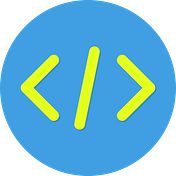# What should I unit test in my code?

## Finding the important and not-so-important parts to test

Hello everyone, in my previous articles, I covered the WHY, and HOW aspects of unit testing. Now is the time to explore the WHAT question.

To be able to unit test our code efficiently, we should know what to test. If we look into assertion libraries, or the APIs provided to write unit test cases, we may feel overwhelmed by the possibilities of test cases that can be written for a piece of code.

While writing efficient test cases serves in the long run (better code quality, preventing regression, help with debugging, etc.), writing excessive test cases can have certain side effects. For starters, they will take longer to run, affecting productivity in one way or the other. Also, there are some scenarios that are essential to test, while some other scenarios not-so-essential to test through unit test cases.

# Essential scenarios

We will explore it through a very basic example (JavaScript code), and see what assertions can be written. The unit test cases are written in Jest.

Let’s say we have an alphanumeric validator method, which takes a string as input and returns a boolean value (true for valid input, false for invalid input).

`export function alphanumericValidator (input?: string) {  if(input is alphanumeric) {  return true;  }  else { return false; }}`

## 1. The common use case scenarios of the implementation

The unit tests should test what the code is supposed to do. The business logic implemented should be tested properly.

For example, we will check if the validator is working properly with below assertions:

`// test if it returns true for valid input//TEST 1: for only alphabets expect(alphanumericValidator('onlyAlphabets')).toBe(true);// TEST 2: for only numbers expect(alphanumericValidator('123')).toBe(true);// TEST 3: for both numeric and alphabets togetherexpect(alphanumericValidator('alphabets123')).toBe(true);`

## 2. Test all the branches/conditions present in the code

All the if and else, switch cases in the code should be unit-tested.

`// TEST 4: testing the else path for invalid inputexpect(alphanumericValidator('alpha123@@@@@@')).toBe(false);// TEST 5: for special characters inputexpect(alphanumericValidator('#./')).toBe(false);`

## 3. Test for unexpected input scenarios

We should test the error scenarios by trying to break the code and verify through test cases that the code does not break at the corner cases or unexpected input scenarios, like null/undefined values. This is especially important when we have optional parameters.

`// TEST 6: for null/undefined input valuesexpect(alphanumericValidator()).toBe(false);expect(alphanumericValidator(null)).toBe(false);`

# Scenarios that can be skipped

## 1. Static values, constants, enumerations, etc

Testing the constants, or enums, or configurations does not bring in much value. Writing test cases for these is pretty much like literally writing

expect(1)toBe(1);

These tests will not help much rather than just increasing the coverage percentage.

## 2. The functionalities of external dependencies or libraries

The term unit testing means to test the unit and hence, testing the actual functionality of any dependency used in that unit does not add any value to the unit testing process. This is because the functionality of that external dependency will already have been verified and tested through separate test cases written while unit testing that unit. Hence, the point.

I am not suggesting, in any case, that these are the thumb rules while writing any test case. Over the years, these are my take on what to include/exclude while unit testing a piece of code. There might be many more points/cases, but I found these points more important and worth sharing.

Note: This article covers some of the what and what-not scenarios for unit testing the code. It is the last article in the series which contains the following:

1. Why should I unit test my code?
2. How should I unit test my code?
2.1. Setup
2.2. Examples

Any feedback is highly appreciable. Thanks for reading, have a great day!!

--

--